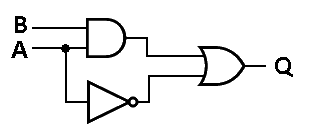# Tutorial - Performing Boolean Algebra inside an FPGA using Look-Up Tables (LUTs)

In the previous article, we discussed the basics of Boolean Algebra, namely how AND, OR, NOT, XOR, and NAND gates work. The concept of truth tables was discussed. In this page, we will expand on this topic of how truth tables work and discuss more complicated Boolean algebra equations.

First it should be noted that all of those discrete logic gates that we discussed previously (AND, OR, etc) actually do not physically exist inside of an FPGA! However it is possible to perform those functions. The way that FPGAs are able to do Boolean algebra is by using Look-Up Tables (LUTs). A Look-Up Table is a discrete block of functionality that can be programmed by the Digital Designer. LUTs use the same truth table concept to relate outputs to inputs. Let's try an example.

Create a truth table for the following Boolean equation: Q = A*B + A'. Perhaps we should define what those symbols mean.

* = AND

+ = OR

' = NOT

^ = XOR

So verbally, the Boolean Equation Q = A*B + A' can be read, "The output Q gets A and B or not A". Let's look at the truth table and the circuit created by this equation. As it can be seen from the image below, it takes three total gates to make this circuit.Truth Table - A*B + A'
Input A Input B Output Q
0 0 1
0 1 1
1 0 0
1 1 1

The truth table in the above example has two inputs (A and B), which means that there are four possible output possibilities. Each input increases the number of possible outputs by a factor of 2. So for one input there are 2 output possibilities, for 2 inputs there are 4 output possibilities, for 3 inputs 8 output possibilities, etc. Mathematically this can be represented by 2^(# of inputs). Let's now look at one more example with three inputs. Here is the equation we are going to create a truth table for: Q = A + (C*B'). Note that the parenthesis indicate that the operation C AND NOT B occurs prior to the OR operation.Truth Table - A + (C*B')
Input A Input B Input C Output Q
0 0 0 0
0 0 1 1
0 1 0 0
0 1 1 0
1 0 0 1
1 0 1 1
1 1 0 1
1 1 1 1As was mentioned in the beginning of this article, discrete logic gates do not actually exist inside of an FPGA. Instead FPGAs use Look-Up Tables or LUTs. The LUT is programmed by the Digital Designer to perform a Boolean algebra equation like the two that we saw above. As you might expect, all possible combinations of boolean expressions need to be able to be programmed into the Look-Up Table. I will say that again a different way: One 3-Input LUT can make any Boolean algebra equation you can think of using 3 input signals. Amazing!

LUTs can come in different sizes depending on the FPGA that you are using, but they all behave the same way. 3-Input LUTs were the norm not too long ago, but today 4-Input and even 5-Input LUTs are common. If you need to make a more complicated expression, you can just use more Look-Up Tables. LUTs are one of the two most fundamental components in an FPGA. A single FPGA has thousands of these components. Now that you are more familiar with these wonderfully versatile components, it is time to discuss the other most important element inside of an FPGA:

The Flip-Flop (AKA Register)

Note to the reader: many textbooks and classrooms will spend a significant amount of time discussing how LUTs can be wired to create the optimal solution to a Boolean expression. Optimal means that it uses the least number of gates possible. Topics such as De Morgan's Law, Karnaugh Maps, the Quine-McCluskey method, and others are usually discussed at great length that describe how to optimize digital logic. However I feel that this is not necessary to begin learning about FPGAs. With the intent of getting you programming an FPGA as soon as possible I will skip these topics. Perhaps some day I will write about them so that if a student needs an outside reference they can read more here, but for now I will stick to what I believe is the minimal amount of knowledge necessary to start with FPGA design.

Help Me Make Great Content!     Support me on Patreon!     Buy a Go Board!